xPlane (mathematics)Overview

In mathematics
Mathematics
Mathematics is the study of quantity, space, structure, and change. Mathematicians seek out patterns and formulate new conjectures. Mathematicians resolve the truth or falsity of conjectures by mathematical proofs, which are arguments sufficient to convince other mathematicians of their validity...

, a plane is a flat, two-dimensional surface
Surface
In mathematics, specifically in topology, a surface is a two-dimensional topological manifold. The most familiar examples are those that arise as the boundaries of solid objects in ordinary three-dimensional Euclidean space R3 — for example, the surface of a ball...

. A plane is the two dimensional analogue of a point
Point (geometry)
In geometry, topology and related branches of mathematics a spatial point is a primitive notion upon which other concepts may be defined. In geometry, points are zero-dimensional; i.e., they do not have volume, area, length, or any other higher-dimensional analogue. In branches of mathematics...

(zero-dimensions), a line
Line (geometry)
The notion of line or straight line was introduced by the ancient mathematicians to represent straight objects with negligible width and depth. Lines are an idealization of such objects...

(one-dimension) and a space
Space
Space is the boundless, three-dimensional extent in which objects and events occur and have relative position and direction. Physical space is often conceived in three linear dimensions, although modern physicists usually consider it, with time, to be part of a boundless four-dimensional continuum...

(three-dimensions). Planes can arise as subspaces of some higher dimensional space, as with the walls of a room, or they may enjoy an independent existence in their own right, as in the setting of Euclidean geometry
Euclidean geometry
Euclidean geometry is a mathematical system attributed to the Alexandrian Greek mathematician Euclid, which he described in his textbook on geometry: the Elements. Euclid's method consists in assuming a small set of intuitively appealing axioms, and deducing many other propositions from these...

.

When working in two-dimensional Euclidean space, the definite article is used, the plane, to refer to the whole space.Unanswered QuestionsHello, friends of good plane mathematics, my problem is: I know some about analytical plane geometry, but I cannot really transmita plane equation ...What is the complete meaning of plane?Encyclopedia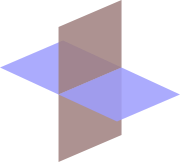In mathematics
Mathematics
Mathematics is the study of quantity, space, structure, and change. Mathematicians seek out patterns and formulate new conjectures. Mathematicians resolve the truth or falsity of conjectures by mathematical proofs, which are arguments sufficient to convince other mathematicians of their validity...

, a plane is a flat, two-dimensional surface
Surface
In mathematics, specifically in topology, a surface is a two-dimensional topological manifold. The most familiar examples are those that arise as the boundaries of solid objects in ordinary three-dimensional Euclidean space R3 — for example, the surface of a ball...

. A plane is the two dimensional analogue of a point
Point (geometry)
In geometry, topology and related branches of mathematics a spatial point is a primitive notion upon which other concepts may be defined. In geometry, points are zero-dimensional; i.e., they do not have volume, area, length, or any other higher-dimensional analogue. In branches of mathematics...

(zero-dimensions), a line
Line (geometry)
The notion of line or straight line was introduced by the ancient mathematicians to represent straight objects with negligible width and depth. Lines are an idealization of such objects...

(one-dimension) and a space
Space
Space is the boundless, three-dimensional extent in which objects and events occur and have relative position and direction. Physical space is often conceived in three linear dimensions, although modern physicists usually consider it, with time, to be part of a boundless four-dimensional continuum...

(three-dimensions). Planes can arise as subspaces of some higher dimensional space, as with the walls of a room, or they may enjoy an independent existence in their own right, as in the setting of Euclidean geometry
Euclidean geometry
Euclidean geometry is a mathematical system attributed to the Alexandrian Greek mathematician Euclid, which he described in his textbook on geometry: the Elements. Euclid's method consists in assuming a small set of intuitively appealing axioms, and deducing many other propositions from these...

.

When working in two-dimensional Euclidean space, the definite article is used, the plane, to refer to the whole space. Many fundamental tasks in mathematics
Mathematics
Mathematics is the study of quantity, space, structure, and change. Mathematicians seek out patterns and formulate new conjectures. Mathematicians resolve the truth or falsity of conjectures by mathematical proofs, which are arguments sufficient to convince other mathematicians of their validity...

, geometry
Geometry
Geometry arose as the field of knowledge dealing with spatial relationships. Geometry was one of the two fields of pre-modern mathematics, the other being the study of numbers ....

, trigonometry
Trigonometry
Trigonometry is a branch of mathematics that studies triangles and the relationships between their sides and the angles between these sides. Trigonometry defines the trigonometric functions, which describe those relationships and have applicability to cyclical phenomena, such as waves...

, graph theory
Graph theory
In mathematics and computer science, graph theory is the study of graphs, mathematical structures used to model pairwise relations between objects from a certain collection. A "graph" in this context refers to a collection of vertices or 'nodes' and a collection of edges that connect pairs of...

and graphing
Graph of a function
In mathematics, the graph of a function f is the collection of all ordered pairs . In particular, if x is a real number, graph means the graphical representation of this collection, in the form of a curve on a Cartesian plane, together with Cartesian axes, etc. Graphing on a Cartesian plane is...

are performed in two-dimensional space, or in other words, in the plane.

## Euclidean geometry

Euclid
Euclid
Euclid , fl. 300 BC, also known as Euclid of Alexandria, was a Greek mathematician, often referred to as the "Father of Geometry". He was active in Alexandria during the reign of Ptolemy I...

set forth the first known axiomatic treatment of geometry. He selected a small core of undefined terms (called common notions) and postulates (or axioms) which he then used to prove various geometrical statements. Although the plane in its modern sense is not directly given a definition anywhere in the Elements, it may be thought of as part of the common notions. In his work Euclid never makes use of numbers to measure length, angle, or area. In this way the Euclidean plane is not quite the same as the Cartesian plane.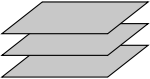## Planes embedded in ℝ3

This section is specifically concerned with planes embedded in three dimensions: specifically, in 3
Cartesian product
In mathematics, a Cartesian product is a construction to build a new set out of a number of given sets. Each member of the Cartesian product corresponds to the selection of one element each in every one of those sets...

.

### Properties

In three-dimensional Euclidean space, we may exploit the following facts that do not hold in higher dimensions:
• Two planes are either parallel or they intersect in a line.
• A line is either parallel to a plane, intersects it at a single point, or is contained in the plane.
• Two lines perpendicular
Perpendicular
In geometry, two lines or planes are considered perpendicular to each other if they form congruent adjacent angles . The term may be used as a noun or adjective...

to the same plane must be parallel to each other.
• Two planes perpendicular to the same line must be parallel to each other.

### Definition with a point and a normal vector

In a three-dimensional space, another important way of defining a plane is by specifying a point and a normal vector to the plane.

Let be the position vector of some known point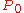in the plane, and let n be a nonzero vector normal to the plane. The idea is that a point with position vector is in the plane if and only if
If and only if
In logic and related fields such as mathematics and philosophy, if and only if is a biconditional logical connective between statements....

the vector drawn fromto is perpendicular to n. Recalling that two vectors are perpendicular if and only if their dot product is zero, it follows that the desired plane can be expressed as the set of all points r such that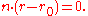(The dot here means a dot product, not scalar multiplication.)
Expanded this becomes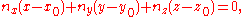which is the familiar equation for a plane.

### Define a plane with a point and two vectors lying on it

Alternatively, a plane may be described parametrically as the set of all points of the form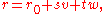where s and t range over all real numbers, and are given vectors defining the plane, and is the vector representing the position of an arbitrary (but fixed) point on the plane. The vectors and can be visualized as vectors starting at and pointing in different directions along the plane. Note that and can be perpendicular, but cannot be parallel.

### Define a plane through three points

Let , , and be non-colinear points.

#### Method 1

The plane passing through , , and can be defined as the set of all points (x,y,z) that satisfy the following determinant
Determinant
In linear algebra, the determinant is a value associated with a square matrix. It can be computed from the entries of the matrix by a specific arithmetic expression, while other ways to determine its value exist as well...

equations: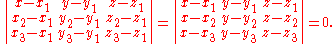#### Method 2

To describe the plane as an equation in the form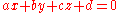, solve the following system of equations: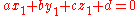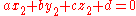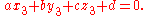This system can be solved using Cramer's Rule
Cramer's rule
In linear algebra, Cramer's rule is a theorem, which gives an expression for the solution of a system of linear equations with as many equations as unknowns, valid in those cases where there is a unique solution...

and basic matrix manipulations. Let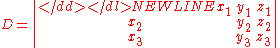.
If D is non-zero (so for planes not through the origin) the values for a, b and c can be calculated as follows: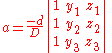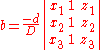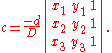These equations are parametric in d. Setting d equal to any non-zero number and substituting it into these equations will yield one solution set.

#### Method 3

This plane can also be described by the "point and a normal vector" prescription above. A suitable normal vector is given by the cross product
Cross product
In mathematics, the cross product, vector product, or Gibbs vector product is a binary operation on two vectors in three-dimensional space. It results in a vector which is perpendicular to both of the vectors being multiplied and normal to the plane containing them...and the point can be taken to be any of the given points , or .

### Distance from a point to a plane

For a plane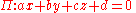and a point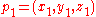not necessarily lying on the plane, the shortest distance from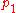to the plane is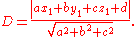It follows thatlies in the plane if and only if
If and only if
In logic and related fields such as mathematics and philosophy, if and only if is a biconditional logical connective between statements....

D=0.

If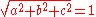meaning that a, b, and c are normalized then the equation becomes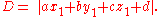### Line of intersection between two planes

The line of intersection between two planes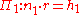and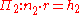where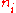are normalized is given by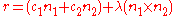where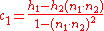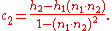This is found by noticing that the line must be perpendicular to both plane normals, and so parallel to their cross product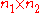(this cross product is zero if and only if the planes are parallel, and are therefore non-intersecting or entirely coincident).

The remainder of the expression is arrived at by finding an arbitrary point on the line. To do so, consider that any point in space may be written as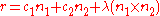, since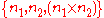is a basis
Basis (linear algebra)
In linear algebra, a basis is a set of linearly independent vectors that, in a linear combination, can represent every vector in a given vector space or free module, or, more simply put, which define a "coordinate system"...

. We wish to find a point which is on both planes (i.e. on their intersection), so insert this equation into each of the equations of the planes to get two simultaneous equations which can be solved for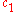and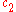.

If we further assume that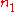and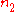are orthonormal then the closest point on the line of intersection to the origin is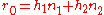. If that is not the case, then a more complex procedure must be used http://mathworld.wolfram.com/Plane-PlaneIntersection.html.

### Dihedral angle

Given two intersecting planes described by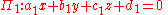and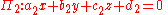, the dihedral angle
Dihedral angle
In geometry, a dihedral or torsion angle is the angle between two planes.The dihedral angle of two planes can be seen by looking at the planes "edge on", i.e., along their line of intersection...

between them is defined to be the anglebetween their normal directions: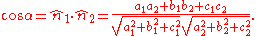## Planes in various areas of mathematics

In addition to its familiar geometric structure, with isomorphism
Isomorphism
In abstract algebra, an isomorphism is a mapping between objects that shows a relationship between two properties or operations.  If there exists an isomorphism between two structures, the two structures are said to be isomorphic.  In a certain sense, isomorphic structures are...

s that are isometries with respect to the usual inner product, the plane may be viewed at various other levels of abstraction
Abstraction (mathematics)
Abstraction in mathematics is the process of extracting the underlying essence of a mathematical concept, removing any dependence on real world objects with which it might originally have been connected, and generalising it so that it has wider applications or matching among other abstract...

. Each level of abstraction corresponds to a specific category
Category (mathematics)
In mathematics, a category is an algebraic structure that comprises "objects" that are linked by "arrows". A category has two basic properties: the ability to compose the arrows associatively and the existence of an identity arrow for each object. A simple example is the category of sets, whose...

.

At one extreme, all geometrical and metric
Metric (mathematics)
In mathematics, a metric or distance function is a function which defines a distance between elements of a set. A set with a metric is called a metric space. A metric induces a topology on a set but not all topologies can be generated by a metric...

concepts may be dropped to leave the topological plane, which may be thought of as an idealized homotopically
Homotopy
In topology, two continuous functions from one topological space to another are called homotopic if one can be "continuously deformed" into the other, such a deformation being called a homotopy between the two functions...

trivial infinite rubber sheet, which retains a notion of proximity, but has no distances. The topological plane has a concept of a linear path, but no concept of a straight line. The topological plane, or its equivalent the open disc, is the basic topological neighborhood used to construct surface
Surface
In mathematics, specifically in topology, a surface is a two-dimensional topological manifold. The most familiar examples are those that arise as the boundaries of solid objects in ordinary three-dimensional Euclidean space R3 — for example, the surface of a ball...

s (or 2-manifolds) classified in low-dimensional topology
Low-dimensional topology
In mathematics, low-dimensional topology is the branch of topology that studies manifolds of four or fewer dimensions. Representative topics are the structure theory of 3-manifolds and 4-manifolds, knot theory, and braid groups. It can be regarded as a part of geometric topology.A number of...

. Isomorphisms of the topological plane are all continuous bijection
Bijection
A bijection is a function giving an exact pairing of the elements of two sets. A bijection from the set X to the set Y has an inverse function from Y to X. If X and Y are finite sets, then the existence of a bijection means they have the same number of elements...

s. The topological plane is the natural context for the branch of graph theory
Graph theory
In mathematics and computer science, graph theory is the study of graphs, mathematical structures used to model pairwise relations between objects from a certain collection. A "graph" in this context refers to a collection of vertices or 'nodes' and a collection of edges that connect pairs of...

that deals with planar graphs, and results such as the four color theorem
Four color theorem
In mathematics, the four color theorem, or the four color map theorem states that, given any separation of a plane into contiguous regions, producing a figure called a map, no more than four colors are required to color the regions of the map so that no two adjacent regions have the same color...

.

The plane may also be viewed as an affine space
Affine space
In mathematics, an affine space is a geometric structure that generalizes the affine properties of Euclidean space. In an affine space, one can subtract points to get vectors, or add a vector to a point to get another point, but one cannot add points. In particular, there is no distinguished point...

, whose isomorphisms are combinations of translations and non-singular linear maps. From this viewpoint there are no distances, but colinearity
Line (geometry)
The notion of line or straight line was introduced by the ancient mathematicians to represent straight objects with negligible width and depth. Lines are an idealization of such objects...

and ratios of distances on any line are preserved.

Differential geometry views a plane as a 2-dimensional real manifold
Manifold
In mathematics , a manifold is a topological space that on a small enough scale resembles the Euclidean space of a specific dimension, called the dimension of the manifold....

, a topological plane which is provided with a differential structure
Differential structure
In mathematics, an n-dimensional differential structure on a set M makes M into an n-dimensional differential manifold, which is a topological manifold with some additional structure that allows us to do differential calculus on the manifold...

. Again in this case, there is no notion of distance, but there is now a concept of smoothness of maps, for example a differentiable or smooth
Smooth function
In mathematical analysis, a differentiability class is a classification of functions according to the properties of their derivatives. Higher order differentiability classes correspond to the existence of more derivatives. Functions that have derivatives of all orders are called smooth.Most of...

path (depending on the type of differential structure applied). The isomorphisms in this case are bijections with the chosen degree of differentiability.

In the opposite direction of abstraction, we may apply a compatible field structure to the geometric plane, giving rise to the complex plane
Complex plane
In mathematics, the complex plane or z-plane is a geometric representation of the complex numbers established by the real axis and the orthogonal imaginary axis...

and the major area of complex analysis
Complex analysis
Complex analysis, traditionally known as the theory of functions of a complex variable, is the branch of mathematical analysis that investigates functions of complex numbers. It is useful in many branches of mathematics, including number theory and applied mathematics; as well as in physics,...

. The complex field has only two isomorphisms that leave the real line fixed, the identity and conjugation.

In the same way as in the real case, the plane may also be viewed as the simplest, one-dimensional (over the complex numbers) complex manifold
Complex manifold
In differential geometry, a complex manifold is a manifold with an atlas of charts to the open unit disk in Cn, such that the transition maps are holomorphic....

, sometimes called the complex line. However, this viewpoint contrasts sharply with the case of the plane as a 2-dimensional real manifold. The isomorphisms are all conformal
Conformal map
In mathematics, a conformal map is a function which preserves angles. In the most common case the function is between domains in the complex plane.More formally, a map,...

bijections of the complex plane, but the only possibilities are maps that correspond to the composition of a multiplication by a complex number and a translation.

In addition, the Euclidean geometry (which has zero curvature
Curvature
In mathematics, curvature refers to any of a number of loosely related concepts in different areas of geometry. Intuitively, curvature is the amount by which a geometric object deviates from being flat, or straight in the case of a line, but this is defined in different ways depending on the context...

everywhere) is not the only geometry that the plane may have. The plane may be given a spherical geometry
Spherical geometry
Spherical geometry is the geometry of the two-dimensional surface of a sphere. It is an example of a geometry which is not Euclidean. Two practical applications of the principles of spherical geometry are to navigation and astronomy....

by using the stereographic projection
Stereographic projection
The stereographic projection, in geometry, is a particular mapping that projects a sphere onto a plane. The projection is defined on the entire sphere, except at one point — the projection point. Where it is defined, the mapping is smooth and bijective. It is conformal, meaning that it...

. This can be thought of as placing a sphere on the plane (just like a ball on the floor), removing the top point, and projecting the sphere onto the plane from this point). This is one of the projections that may be used in making a flat map of part of the Earth's surface. The resulting geometry has constant positive curvature.

Alternatively, the plane can also be given a metric which gives it constant negative curvature giving the hyperbolic plane
Hyperbolic geometry
In mathematics, hyperbolic geometry is a non-Euclidean geometry, meaning that the parallel postulate of Euclidean geometry is replaced...

. The latter possibility finds an application in the theory of special relativity
Special relativity
Special relativity is the physical theory of measurement in an inertial frame of reference proposed in 1905 by Albert Einstein in the paper "On the Electrodynamics of Moving Bodies".It generalizes Galileo's...

in the simplified case where there are two spatial dimensions and one time dimension. (The hyperbolic plane is a timelike hypersurface
Hypersurface
In geometry, a hypersurface is a generalization of the concept of hyperplane. Suppose an enveloping manifold M has n dimensions; then any submanifold of M of n − 1 dimensions is a hypersurface...

in three-dimensional Minkowski space
Minkowski space
In physics and mathematics, Minkowski space or Minkowski spacetime is the mathematical setting in which Einstein's theory of special relativity is most conveniently formulated...

.)

## Topological and differential geometric notions

The one-point compactification of the plane is homeomorphic to a sphere
Sphere
A sphere is a perfectly round geometrical object in three-dimensional space, such as the shape of a round ball. Like a circle in two dimensions, a perfect sphere is completely symmetrical around its center, with all points on the surface lying the same distance r from the center point...

(see stereographic projection
Stereographic projection
The stereographic projection, in geometry, is a particular mapping that projects a sphere onto a plane. The projection is defined on the entire sphere, except at one point — the projection point. Where it is defined, the mapping is smooth and bijective. It is conformal, meaning that it...

); the open disk is homeomorphic to a sphere with the "north pole" missing; adding that point completes the (compact) sphere. The result of this compactification is a manifold
Manifold
In mathematics , a manifold is a topological space that on a small enough scale resembles the Euclidean space of a specific dimension, called the dimension of the manifold....

referred to as the Riemann sphere
Riemann sphere
In mathematics, the Riemann sphere , named after the 19th century mathematician Bernhard Riemann, is the sphere obtained from the complex plane by adding a point at infinity...

or the complex projective line
Projective line
In mathematics, a projective line is a one-dimensional projective space. The projective line over a field K, denoted P1, may be defined as the set of one-dimensional subspaces of the two-dimensional vector space K2 .For the generalisation to the projective line over an associative ring, see...

. The projection from the Euclidean plane to a sphere without a point is a diffeomorphism
Diffeomorphism
In mathematics, a diffeomorphism is an isomorphism in the category of smooth manifolds. It is an invertible function that maps one differentiable manifold to another, such that both the function and its inverse are smooth.- Definition :...

and even a conformal map
Conformal map
In mathematics, a conformal map is a function which preserves angles. In the most common case the function is between domains in the complex plane.More formally, a map,...

.

The plane itself is homeomorphic (and diffeomorphic) to an open disk
Disk (mathematics)
In geometry, a disk is the region in a plane bounded by a circle.A disk is said to be closed or open according to whether or not it contains the circle that constitutes its boundary...

. For the Lobachevsky plane such diffeomorphism is conformal, but for the Euclidean plane it is not.

## See also

• Half-plane
• Hyperplane
Hyperplane
A hyperplane is a concept in geometry. It is a generalization of the plane into a different number of dimensions.A hyperplane of an n-dimensional space is a flat subset with dimension n − 1...

• Line-plane intersection
Line-plane intersection
In analytic geometry, the intersection of a line and a plane can be the empty set,a point, ora line. Distinguishing these cases, and determining equations for the point and line in the latter cases have use, for example, in computer graphics, motion planning, and collision detection.-Parametric...

• Plane of rotation
Plane of rotation
In geometry, a plane of rotation is an abstract object used to describe or visualise rotations in space. In three dimensions it is an alternative to the axis of rotation, but unlike the axis of rotation it can be used in other dimensions, such as two, four or more dimensions.Mathematically such...

• Point on plane closest to origin
• Projective plane
Projective plane
In mathematics, a projective plane is a geometric structure that extends the concept of a plane. In the ordinary Euclidean plane, two lines typically intersect in a single point, but there are some pairs of lines that do not intersect...

## External links

The source of this article is wikipedia, the free encyclopedia.  The text of this article is licensed under the GFDL.